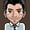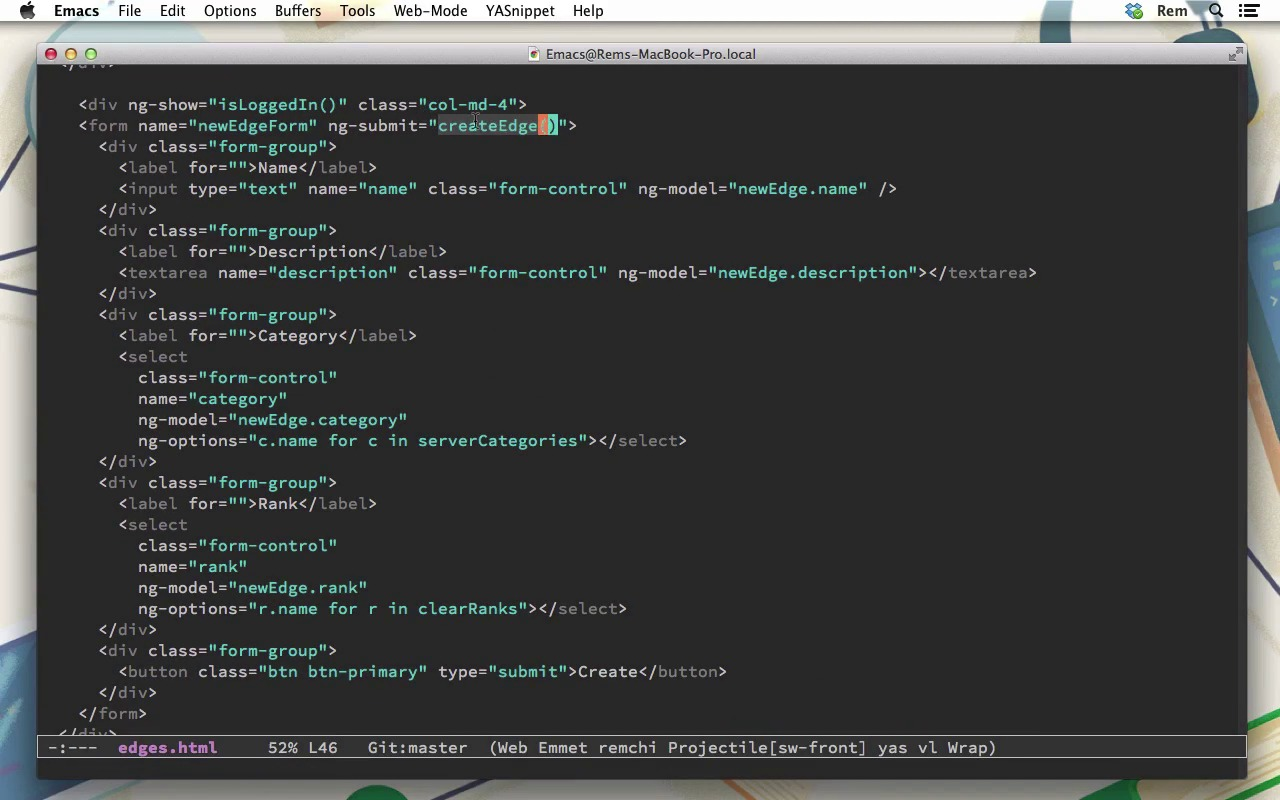7 days of WordPress plugins, themes & templates - for free!* Unlimited asset downloads! Start 7-Day Free TrialFREELessons: 16Length: 3 hours• Overview
• Transcript

# 4.3 Create New Edge

In this lesson we'll let authenticated users create new edges.

## 5.Conclusion1 lesson, 00:31

### 4.3 Create New Edge

Hi. So, now that we can log in. Let's implement creation of new edges. But first, let's create several categories in a database. So let's go to terminal, and to console. And from console let's create Category with name one, with name hello and with name awesome. Okay, so now we have categories, and now we need to fetch these categories. Because right now on Edges page, categories are hard-coded in JavaScript. But we want to fetch them and populate them right here. So they will be populated from server. Ideally, we want this same behavior for Rank. But we'll skip it for Rank in this course. Okay, so let's go to editor into the EdgesController. And right here we fetch Edges from server. And now we need to do the same thing for categories. So, let's go to category service. Right here, we have just this query so let's delete it. And instead of service, we'll use factory. And we will have the resource. So this resource will return resource, api categories. So now in routes.rb file, we need to define another route here. And that's gonna be categories only index. And now we need to create categories controller. So very basic stuff. In api we create categories_controller.rb. So inside of this controller, we define index section which renders json Category.all and root false. So, let's save it and go back to browser. Now we can see that we have no categories at all. So, let's reload the page, and now we can see that we have actual categories one, hello, and awesome. Excellent, but we also want to have here all option. So let's go back to our edges.js, and I'm gonna just make some white space here. So, for that, let's name it as serverCategories. Okay, so when we fetch them, we also will have simple categories, and these simple categories will be just an array of one particular object name with All. And now when categories.query is finished, we'll make this a call back. And inside of it, we'll set scope.categories to scope.categories.concat. So we concatenate two arrays. The first one here with just a name All, and the second one is a serverCategories. So we do scope.serverCategories, like this. So let's save it, go back to browser, and now you can see that we have all options. Excellent. So, now let's go to edges.html file. Okay, so now here in our edges.html we need to make some small adjustments, because, basically, we want, on the left, we want this edges with filters and edges list. And on the right we want to have new edges form. So, to make it, we have here the class row. And then inside of this, we will have div with class col-md-8. So in here I change 3 to 4 because there are only 12 columns. I remove this one from here, paste it here, and add another div. Let's indent them. And id edges as well will have the class col-md-12. Okay. So basically we have a row and two columns of this row 8 in which we have filters and edges. And here we'll have div with our form which also has column 4, and then we just close all of these tags. Let's Save it. And if we go to the browser, we do not see any changes at all because we are not logged in. So let's log in. [BLANK_AUDIO] And now we can see the changes, but that's not actually what we want. Okay, so here of course I need to have the closing div. Okay, so now it's better. So here we have Edges, and here we have the new form. So let's have a look what we have in this new form. So it's just a simple form with the name of newEdgeForm and this submit of createEdge. And then we have the name. And we bind it to the ng-model newEdge with a name. Then we have the description and to select elements, Category which depends on serverCategories. And rank which depends on clearRanks. Because we also have ranks as you can see here with All. But when we create them, we do not want to have All. So, for example here, Categories just have one, hello, and awesome without this All options. And we do not have ranks yet. So, let's add ranks here. So, we go to edges.js. And we have ranks here, so let's create scope.clearRanks. And for that we just take scope.ranks and slice it from the first element. So now if you go back to browser, we'll see that ranks are here, Novice and Seasoned. Excellent. So now, let's create new edge. For that, on scope, let's define newEdge model. And we just instantiate new Edges service. So, this service is a resource. So when we instantiate it, we instantiate the resource. And now, we need to create createEdge function. We just a function. And inside of it we're going to make this scope.newEdge.save. And that's the method provided to us by resource service. So if we do it right now, let's Save it and go to browser. And I'm going to open here, Edges is not defined. Okay, my bad, edges like this. So now if you go back here and click on Create, you can see that we have api Edges request, and there's the post request to api Edges. So, so resource service was created for rest APIs. Okay, good. So here we will have edge, and that's a promise, so we do then and a simple call back. And this call back, we want first of all to push to the edges collection response from the server. Okay, so here we have the response. And after that, again on scope, we want to redefine newEdge as new edges. So this way, we reset form to initial state. So let's Save it. And now we need to implement backend. So for that, we go to routes, first of all, and in edges we add here, create action. Save it. Now we go to EdgesController, and here let us define create action. And we're going to be as primitive as possible. So first of all we're going to create rank, and that's a requirement. And we're going to create it with mode rank and value params rank name. So how do I know it? Okay, so if we go back to browser, let's make some request with data actually, so we making the request. And now you we can see here we're making the request with description name, with category as an object, and with rank as an object with a name. So that's where I take this rank name. So now that I have rank created, I can create edge. So we do Edge.new. And we do all this name equals params name. Description equals params description. Category_id equals params category id. Requirements equals array of rank, we have only one requirement now. And after this, we just Save it. And render json this edge object, with root to false. Okay, and that will do it. So let's have a look. Let's reload the page and try to create new edge. So, with Category one and Rank Novice. And when we create it, you can see that this edge has been here in our list, and the form has been cleared out. And of course if you reload the page, it's still there because now it's in the database. And we can create another one. And let's do another Category awesome, and Seasoned. And of course our Category filter works as it should work, and all other filters works as well. Okay, in this episode we implemented creation of a new edge functionality, and we use for this Angular resource. So thank you very much for your time, and see you in the next episode.

Back to the top